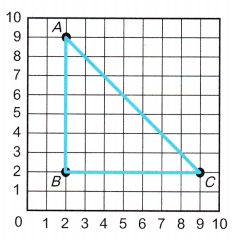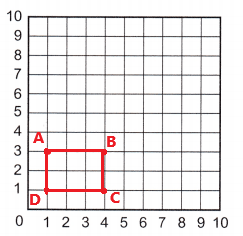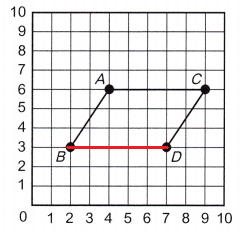# McGraw Hill Math Grade 5 Chapter 11 Lesson 5 Answer Key Analyzing Shapes and Lines

All the solutions provided in McGraw Hill Math Grade 5 Answer Key PDF Chapter 11 Lesson 5 Analyzing Shapes and Lines are as per the latest syllabus guidelines.

## McGraw-Hill Math Grade 5 Answer Key Chapter 11 Lesson 5 Analyzing Shapes and Lines

Question 1.
On the grid, draw lines $$\overline{A B}$$, $$\overline{B C}$$, and $$\overline{C A}$$. What shape did you draw?
____________
What are the coordinates of the shape?Explanation:
After drawing the given lines $$\overline{A B}$$, $$\overline{B C}$$, and $$\overline{C A}$$ on the grid the shape formed with these lines is triangle.
The coordinates of the triangle are A (2, 9) , B (2, 2), C (9, 2).

Question 2.
Draw a rectangle on the grid. Be sure each corner is a point Label the points.
What are the coordinates of the points you drew?Explanation:
A rectangle is drawn on the grid and labelled the points as A, B, C, D.
The coordinates of the rectangle are A (1, 3), B (4, 3), C (4, 1) and D (1, 1).

Question 3.
Kim is building a flower box. She draws lines $$\overline{A B}$$, $$\overline{C D}$$, and $$\overline{A C}$$. She wants to draw a fourth side parallel to $$\overline{A C}$$. Draw the line.
What shape is the flower box?Kim drawn a lines $$\overline{A B}$$, $$\overline{C D}$$, and $$\overline{A C}$$. She wants to draw a fourth side parallel to $$\overline{A C}$$. After drawing the fourth side the shape of the flower box is parallelogram.